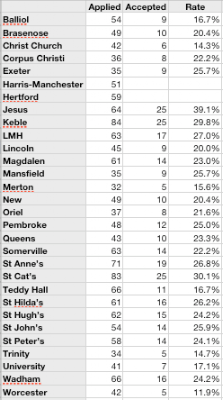## Friday, December 10, 2010

### Are some Oxford colleges racist?

As a follow up to yesterday's post about statistics on black students applying to and being accepted by Oxbridge colleges and thought I'd follow up on the "Merton problem". In the original article the author writes: "Merton College, Oxford, has not admitted a single black student for five years".

Two questions come out from that. First, how likely is the event "A single Oxford college doesn't admit a single black student for five years in a row" and secondly, "are some Oxford colleges racist?".

In an email from Ben Goldacre the first question is answered. Ben calculates the p-value of the "Merton problem" as (0.29^5) * 38 = 0.0779423662. i.e. given the small number of black students applying to Oxford you can't with statistical confidence say that the "Merton problem" is anything more than a natural consequence of randomness.

So, let's turn to the second question. Happily, the article author has published the documents he received from Oxford and Cambridge and from them we can calculate the rate at which black students are accepted at each of the colleges (for some reason there's no acceptance data for Hertford and Harris-Manchester).

Here's the data:There I've shown just the total number of black students applying to each college from 1999 to 2009, the total number accepted and calculated an acceptance rate. You'll notice that some colleges get more applicants than other. Interestingly, Merton received the lowest number of black applicants which goes along way to understanding why they didn't accept more students.

Now, let's turn to the acceptance rate. Do the acceptance rates tell us that some colleges are more racist than others? For the statistical test take the null hypothesis as "black students are accepted at the same rate by all Oxford colleges" (i.e. what I'm asking the test is "does this data look like it's not uniformly distributed?").

Using my old friend the chi-square test we get a value of 39.161 for 27 degrees of freedom (there are 28 colleges with data). Looking that up in a chi-square table gives p-values of 40.11 (p=0.05), 46.96 (p=0.01) and 55.48 (p=0.001). That means we can't reject the null hypothesis. This data doesn't give us evidence that the acceptance rates at Oxford colleges are anything other than uniform.

#### 3 comments:

Gareth Bebb said...

Hi John,
I'm Jesus alumni and was very shocked to see your figures on the college. Your table suggests a 39% (highest) acceptance rate for black students- the FOI table puts it at 7.8% (lowest) by my calculations. Don't know whether this affects your conclusion and I'm sure it's a genuine input error but does somewhat invalidate your analysis.

John Graham-Cumming said...

Gareth.

I stand corrected on the Jesus figure. Clearly some fat-fingered typing on my part.

With the correct figure the chi square value is 37.211 which doesn't change the conclusion. Still not statistically significant.

Although, if I was Jesus college I would be asking myself why I'm the lone college in that position.

John.Anonymous said...

Aside from statistics, it's also just not true that Merton didn't accept any black students in the past five years. It did, but not every student discloses ethnicity on forms.